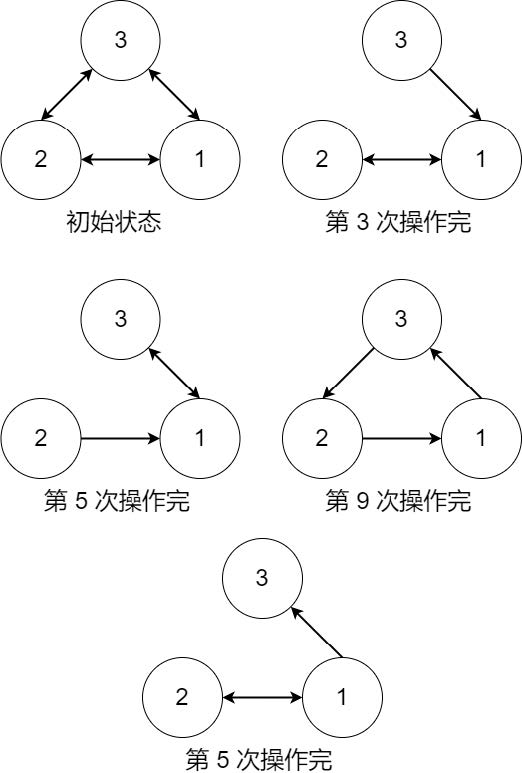# #775. 【CSP-S 2022】星战

1. 敌人会摧毁某个虫洞，这会使它连接的两个据点无法再通过这个虫洞直接到达，但这样的打击无法摧毁它连接的两个据点。
2. 敌人会摧毁某个据点，由于虫洞的主要技术集中在出口处，这会导致该据点的所有还未被摧毁的虫洞被一同摧毁。而从这个据点出发的虫洞则不会摧毁

• A 型特种部队则可以将某个特定的虫洞修复。
• B 型特种部队可以将某据点的所有损坏的虫洞修复。

• 如果从我方的任何据点出发，在选择了合适的路线的前提下，可以进行无限次的虫洞穿梭（可以多次经过同一据点或同一虫洞），那么这个据点就可以实现反击
• 为了使虫洞穿梭的过程连续，尽量减少战舰在据点切换虫洞时的质能损耗，当且仅当只有一个从该据点出发的虫洞可用时，这个据点可以实现连续穿梭
• 如果我方所有据点都可以实现反击，也都可以实现连续穿梭，那么这个时刻就是一个绝佳的反攻时刻。

### 输入格式

• 若 $t = 1$，接下来两个整数 $u, v$ 表示敌人摧毁了从据点 $u$ 出发到据点 $v$ 的虫洞。保证该虫洞存在且未被摧毁。
• 若 $t = 2$，接下来一个整数 $u$ 表示敌人摧毁了据点 $u$。如果该据点的虫洞已全部 被摧毁，那么这次袭击不会有任何效果。
• 若 $t = 3$，接下来两个整数 $u, v$ 表示我方修复了从据点 $u$ 出发到据点 $v$ 的虫洞。保证该虫洞存在且被摧毁。
• 若 $t = 4$，接下来一个整数 $u$ 表示我方修复了据点 $u$。如果该据点没有被摧毁的虫洞，那么这次修复不会有任何效果。

### 样例一

#### input

3 6
2 3
2 1
1 2
1 3
3 1
3 2
11
1 3 2
1 2 3
1 1 3
1 1 2
3 1 3
3 3 2
2 3
1 3 1
3 1 3
4 2
1 3 2

#### output

NO
NO
YES
NO
YES
NO
NO
NO
YES
NO
NO

#### explanation### 限制与约定

$1 \sim 3$ $10$ $20$ $50$
$4 \sim 8$ ${10}^3$ ${10}^4$ ${10}^3$
$9 \sim 10$ $5 \times {10}^5$ $5 \times {10}^5$ $5 \times {10}^5$ 保证没有 $t = 2$ 和 $t = 4$ 的情况
$11 \sim 12$ 保证没有 $t = 4$ 的情况
$13 \sim 16$ ${10}^5$
$17 \sim 20$ $5 \times {10}^5$